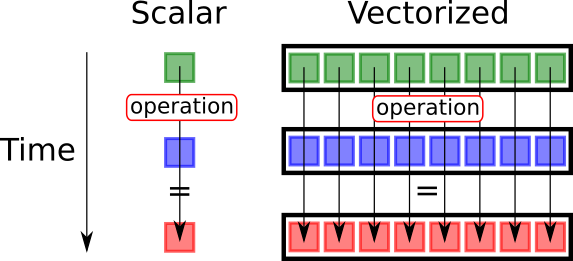### 6.6.1 : What is vectorization ?

It is a copy-paste of the computing part of a CPU (ALU) to enable several computing at the same time.Comparison between scalar (classical) computation and vectorization.

Different vectorized architectures exist :
• SSE2 : compute 2 floating point single precision computation at the same time
• SSE4 : compute 4 floating point single precision computation at the same time
• AVX : compute 8 floating point single precision computation at the same time
• AVX 512 : compute 16 floating point single precision computation at the same time